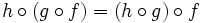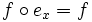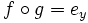# Groupoid

(diff) ← Older revision | Latest revision (diff) | Newer revision → (diff)
This is a variation of group|Find other variations of group | Read a survey article on varying group

## Definition

### Definition in terms of categories

A groupoid is a (locally small) category where every morphism is invertible.

### Definition in basic terms

A groupoid is the following:

• A collection$X$ of points.
• A collection$P$ of paths$f$, with two maps$s:P \to X$ and$t:P \to X$, termed the source and terminus maps. Denote by$P(x,y)$ the paths with source$x$ and terminus$y$.
• For points$x,y,z \in X$, a map$\circ : P(y,z) \times P(x,y) \to P(x,z)$.

satisfying the following:

• Associativity: For every$x,y,z,w \in X$, and every$f \in P(x,y), g \in P(y,z), h \in P(z,w)$,$h \circ (g \circ f) = (h \circ g) \circ f$.
• Identity element: For every$x \in X$, there exists a map$e_x \in P(x,x)$ such that$e_x \circ f = f$ for all$f$ with$t(f) = x$, and$f \circ e_x = f$ for all$f$ with$s(f) = x$.
• Inverses: For every$f \in P(x,y)$, there exists a$g \in P(y,x)$ such that$f \circ g = e_y$ and$g \circ f = e_x$. Such a$g$ is denoted by$f^{-1}$.

### Further term:connected

A connected groupoid is a groupoid where$P(x,y)$ is nonempty for any points$x,y \in X$.

## Particular cases

• For any groupoid, the paths from any point to itself form a group under composition.
• The notion of groupoid with one point is equivalent to the notion of group, where the group is simply all the paths from that point to itself under composition.
• If there is a path from$x$ to$y$, the group of paths from$x$ to itself is isomorphic to the group of paths from$y$ to itself. Further, the isomorphism is uniquely specified up to (possibly) conjugation in the source group (or equivalently, up to conjugation in the target group).
• Building on the above, we get a homomorphism from automorphism group of connected groupoid to outer automorphism group at a point.